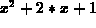Next: Maple at WPI Up: Getting Started With Previous: Getting Started With

## Introduction

The software package Maple is an example of a Computer Algebra System (CAS for short), meaning that it is capable of dealing with problems in symbolic form. This is in contrast to the numerical approach that is usually involved in programming computers to solve problems. A CAS is capable of understanding functions and expressions, but can also be used to perform algebraic manipulations with expressions and functions. For example, if you give a CAS an expression likeit understands that x is a variable and Maple can do things like evaluate the expression at a given value of x, factor the expression, substitute another expression in for x, multiply two expressions together, and more - all without making mistakes and much more rapidly than you can do them by hand. Maple also will let you define your own functions, which can be plotted, manipulated, combined with other functions, etc. Maple will also perform many of the manipulations that usually occupy a major part of a calculus course - differentiation, integration, substitution, solving differential equations, etc. On the other hand, we will run into some situations where Maple doesn't do exactly what you would expect. That is, Maple is not perfect - it has some bugs in it. Fortunately, there aren't many of these!

Maple is a powerful tool that can free you from a lot of calculational drudgery, but there is a catch. To use it properly, you have to understand the rules of the game. These rules can be divided into two parts: rules for Maple syntax, i.e. what are the commands that Maple understands and how must you express them, and rules of mathematics. Maple can perform calculations at blinding speed, but you are responsible for making those calculations meaningful and mathematically correct. In this section, we will concentrate on the rules for Maple syntax.Next: Maple at WPI Up: Getting Started With Previous: Getting Started With

William W. Farr
Mon Aug 28 09:31:56 EDT 1995